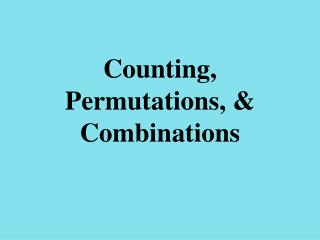DownloadDownload PresentationCounting, Permutations, & Combinations

# Counting, Permutations, & Combinations

Télécharger la présentation## Counting, Permutations, & Combinations

- - - - - - - - - - - - - - - - - - - - - - - - - - - E N D - - - - - - - - - - - - - - - - - - - - - - - - - - -
##### Presentation Transcript

1. Counting, Permutations, & Combinations

2. A counting problem asks “how many ways” some event can occur. Ex. 1: How many three-letter codes are there using letters A, B, C, and D if no letter can be repeated? One way to solve is to list all possibilities.

3. Ex. 2: An experimental psychologist uses a sequence of two food rewards in an experiment regarding animal behavior. These two rewards are of three different varieties. How many different sequences of rewards are there if each variety can be used only once in each sequence? Next slide

4. Another way to solve is a factor tree where the number of end branches is your answer.

5. Fundamental Counting Principle Suppose that a certain procedure P can be broken into n successive ordered stages, S1, S2, . . . Sn, and suppose that S1 can occur inr1 ways. S2 can occur inr2 ways. Sn can occur inrn ways. Then the number of ways P can occur is

6. Ex. 2: An experimental psychologist uses a sequence of two food rewards in an experiment regarding animal behavior. These two rewards are of three different varieties. How many different sequences of rewards are there if each variety can be used only once in each sequence? Using the fundamental counting principle: 3 2

7. Permutations An r-permutation of a set of n elements is an orderedselection of r elements from the set of n elements ! means factorial Ex. 3! = 3∙2∙1 0! = 1

8. Ex. 1:How many three-letter codes are there using letters A, B, C, and D if no letter can be repeated? Note: The order does matter

9. Combinations The number of combinations of n elements taken r at a time is Order does NOT matter! Where n & r are nonnegative integers & r<n

10. Ex. 3: How many committees of three can be selected from four people? Use A, B, C, and D to represent the people Note: Does the order matter?

11. Ex. 4: How many ways can the 4 call letters of a radio station be arranged if the first letter must be W or K and no letters repeat?

12. Ex. 5: In how many ways can our class elect a president, vice-president, and secretary if no student can hold more than one office?

13. Ex. 7: Given the digits 5, 3, 6, 7, 8, and 9, how many 3-digit numbers can be made if the first digit must be a prime number? (can digits be repeated?) Think of these numbers as if they were on tiles, like Scrabble. After you use a tile, you can’t use it again.

14. Ex. 9: Suppose there are 15 girls and 18 boys in a class. In how many ways can 2 girls and 2 boys be selected for a group project? 15C2 X 18C2 = 16,065